# Hilbert Space

Also found in: Dictionary, Thesaurus, Wikipedia.
Related to Hilbert Space: vector space, Banach space

## Hilbert space

[′hil·bərt ¦spās]
(mathematics)
A Banach space which also is an inner-product space with the inner product of a vector with itself being the same as the square of the norm of the vector.

## Hilbert Space

a mathematical concept extending Euclidean space to one of infinite dimensions. The concept arose at the turn of the 20th century in the form of a natural logical conclusion from the papers of the German mathematician D. Hilbert as a result of the generalization of the facts and methods related to the expansion of functions into orthogonal series and to the study of integral equations. Developing gradually, the concept of Hilbert space found increasingly wider applications in various branches of mathematics and theoretical physics. The concept is one of the most important in mathematics.

Originally, Hilbert space denoted the space of sequences with the converging series of squares (the so-called l2 space). The elements (vectors) of such a space are the infinite numerical sequences

x = (x1, x2, … , xn, …)

such that the series x12 + x22 + … + xn2 + … converges. The sum of the two vectors x + y and the vector λx, where λ is a real number, are determined naturally:

x + y = (x1 + y1, …, xn + yn, …)

λx = (λx1, λx2, … , λxn, …)

For any vectors x, y ∈ l2, the equation

(x, y) = x1y1 + x2y2 + … + xn yn + … )

determines their scalar product, and the length (norm) of vector x is understood to mean the nonnegative number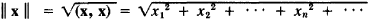The scalar product is always finite and satisfies the inequality │ (x, y) │ ≤ ║ x ║ ║ y ║. The sequence of vectors xn is said to be converging to vector x if ║ xn − x ║ → 0 as n → ∞. Many of the definitions and facts concerning the theory of finite-dimensional Euclidean spaces also apply to Hilbert space. For example, the equation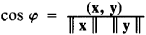where 0 ≤ϕ ≤ π, determines the angle between vectors x and y. Two vectors x and y are said to be orthogonal if (x, y) = 0. The l2 space is complete: every fundamental Cauchy sequence of the elements of this space (that is, the sequence xn satisfying the condition ║ xn - xm ║ → 0 as n, m → ∞) has a limit. In contrast to Euclidean spaces, the l2 Hilbert space is infinite dimensional, that is, infinite systems of linearly independent vectors exist in this space. For example, such a system is formed by the unit vectors

e1 = (1, 0, 0, …), e2 = (0, 1, 0,…), …

In this case, for any vector x from l2, the expansion

(1) x = x1e1 + x2e2 + …

applies in the system (en).

Another important example of Hilbert space is the L2 space of all measurable functions defined within a certain interval [a,b] for which the integral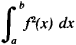is finite. This integral is to be understood as an integral in the Lebesgue sense. In this case, the functions differing from each other only by the set of measure zero are regarded as identical. Multiplication of functions by a number and their addition are performed in the usual manner, and the scalar product is understood to mean the integral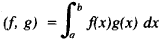In this case the norm is equal to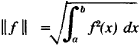The role of the unit vectors in the preceding example may be played by any functions φi(x) from L2 possessing the properties of orthogonalityand normalization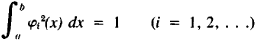as well as the following property of completeness: if f(x) belongs to L2 andthen fx = 0 everywhere, except for the set of measure zero. Over the interval [0, 2π], such a system of functions may be represented by the trigonometric systemExpansion (1) corresponds to the expansion of function f(x) from L2 into the Fourier serieswhich converges to f(x) by the norm of the L2 space. In this case, for every function f(x), the Parseval equality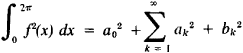is fulfilled.

The correspondence between the functions f(x) from L2 and the sequences of their Fourier coefficients a0, a1, b1, a2, b2, … is a mutually single-valued mapping of L2 onto l2, preserving the operations of addition and multiplication by numbers as well as the lengths and scalar products. Thus, these spaces are isomorphous and isometric and, hence, have the same structure.

In a broader sense, Hilbert space is understood to mean an arbitrary linear space in which the scalar product is given and which is complete with respect to the norm generated by this scalar product. Depending on whether multiplication is defined only by real numbers for the elements of the Hilbert space H or the elements of H may be multiplied by arbitrary complex numbers, real and complex Hilbert spaces are distinguished. In the latter case, the scalar product is understood to mean the complex function (x, y) defined for any pair x, y of elements from H and possessing the following properties:

(1) (x, x) = 0 only and only if x = 0,

(2) (x, x) ≥ 0 for any x from H,

(3) (x + y, z) = (x, z) + (y, z),

(4) (λx, y) = λ(x, y) for any complex number λ, and

(5) (x, y) =, where the bar denotes a complex conjugate quantity.

The norm of element x is determined by the equality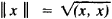Complex Hilbert spaces play a larger role in mathematics and its applications than real Hilbert spaces. One of the most important trends in the theory of Hilbert spaces is the study of linear operators in Hilbert space. It is precisely this problem area that is connected with the numerous applications of Hilbert space in the theory of differential and integral equations, probability theory, quantum mechanics, and other areas.

### REFERENCES

Kolmogorov, A. N., and S. V. Fomin. Elementy teorii funktsii i funktsional’nogo analiza, 2nd ed. Moscow, 1968.
Liusternik, L. A., and V. I. Sobolev. Elementy funktsional’nogo analiza, 2nd ed. Moscow, 1965.
Dunford, N., and J. Schwartz. Lineinye operatory. Vol. 1: Obshchaia teoria. Moscow, 1962. (Translated from English.)
Day, M. M. Normirovannye lineinye prostranstva. Moscow, 1961., (Translated from English.)

IU. V. PROKHOROV

References in periodicals archive ?
An Introduction to Hilbert Space, Chelsea Press, New York, 114 pp.
Describing the evolution not of quantum states, but of determinate properties, these dynamics amount to an admission that the fundamental state space of the theory is not Hilbert space but a space of ordered pairs of Hilbert-space states and determinate-property states.
the set SPN, or the set containing position and momentum--is in one-one correspondence with the set S(H) of closed subspaces of the Hilbert space H in which the given class of observables is represented.
maps due to Wittstock (Haagerup [H2] and Paulsen proved it independently, see [Pal])says that there is a Hilbert space H, a representation [pi] : B([l.
As a point of Hilbert space representing ad finitum the "quantum state" of an entire narrative system, his indeterminacy is then indistinguishable from the causeless effect and the effectless cause of that state, which is now a story that has Merv Griffm in it but which determines how it shall be read, from a point outside it, his ever shifting cachet as a performance artist in late capitalist showbiz.
Disk-Cyclicity Criterion for tuples) Let H be a separable infinite dimensional Hilbert space and T = ([T.
Luke, "A strongly convergent reflection method for finding the projection onto the intersection of two closed convex sets in a Hilbert space," Journal of Approximation Theory, vol.
s], s > 0, are part of a Hilbert scale, and Y is a Hilbert space.
t] is R(G) [cross product] B(K), where K is any Hilbert space of finite dimension [dim.
In particular, the 4-current is related to a construction of a Hilbert space.

Site: Follow: Share:
Open / Close